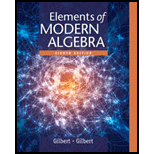# In each of the following parts, a relation R is defined on the set Z of all integers. Determine in each case whether or not R is reflexive, symmetric or transitive. Justify your answers. a. x R y if and only if x = 2 y . b. x R y if and only if x = − y . c. x R y if and only if y = x k for some k in Z . d. x R y if and only if x &lt; y . e. x R y if and only if x ≥ y . f. x R y if and only if x = | y | . g. x R y if and only if | x | ≤ | y + 1 | . h. x R y if and only if x y ≥ 0 . i. x R y if and only if x y ≤ 0 . j. x R y if and only if | x − y | = 1 . k. x R y if and only if | x − y | &lt; 1 .### Elements Of Modern Algebra

8th Edition
Gilbert + 2 others
Publisher: Cengage Learning,
ISBN: 9781285463230### Elements Of Modern Algebra

8th Edition
Gilbert + 2 others
Publisher: Cengage Learning,
ISBN: 9781285463230

#### Solutions

Chapter
Section
Chapter 1.7, Problem 2E
Textbook Problem

## Expert Solution

### Want to see the full answer?

Check out a sample textbook solution.See solution

### Want to see this answer and more?

Experts are waiting 24/7 to provide step-by-step solutions in as fast as 30 minutes!*

See Solution

*Response times vary by subject and question complexity. Median response time is 34 minutes and may be longer for new subjects.